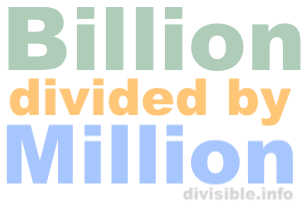50 billion divided by 350 millionHere we will show you how to calculate 50 billion divided by 350 million. One billion is one thousand times larger than one million. 50 billion is 50 followed by nine zeros, and 350 million is 350 followed by six zeros:

50 billion
= 50,000,000,000

350 million
= 350,000,000

You may be able to type 50,000,000,000 divided by 350,000,000 into your calculator, but because of all the zeros it is easy to make a mistake, and many calculators do not allow you to enter that many digits.

To make it easier, remove the last six zeros of each number. Then, 50,000,000,000 divided by 350,000,000 becomes this:

50,000 divided by 350

Now, when we enter 50,000 ÷ 350 into our calculator, we get the answer to 50 billion divided by 350 million as follows:

≈ 142.8571

Billion divided by Million Calculator
Use this calculator to divide any amount of billions by millions.

What is  billion divided by  million?

What is 50 billion divided by 355 million?
Here is the next billion divided by million problem in our database.